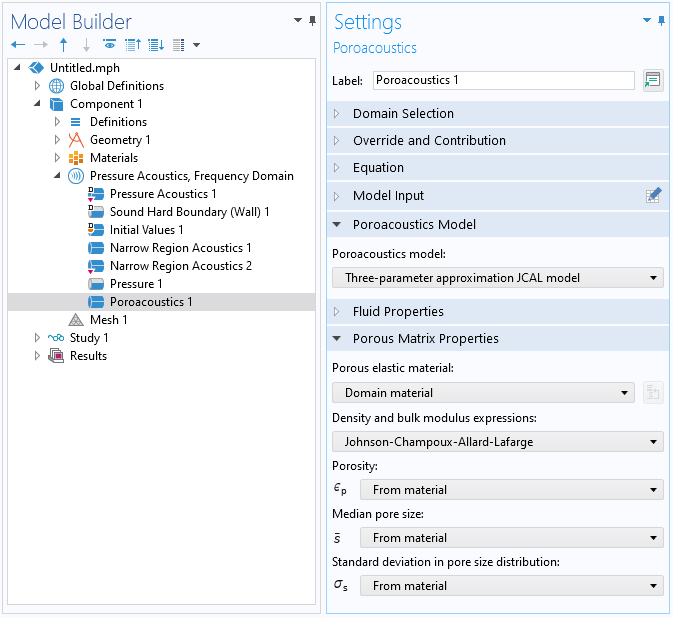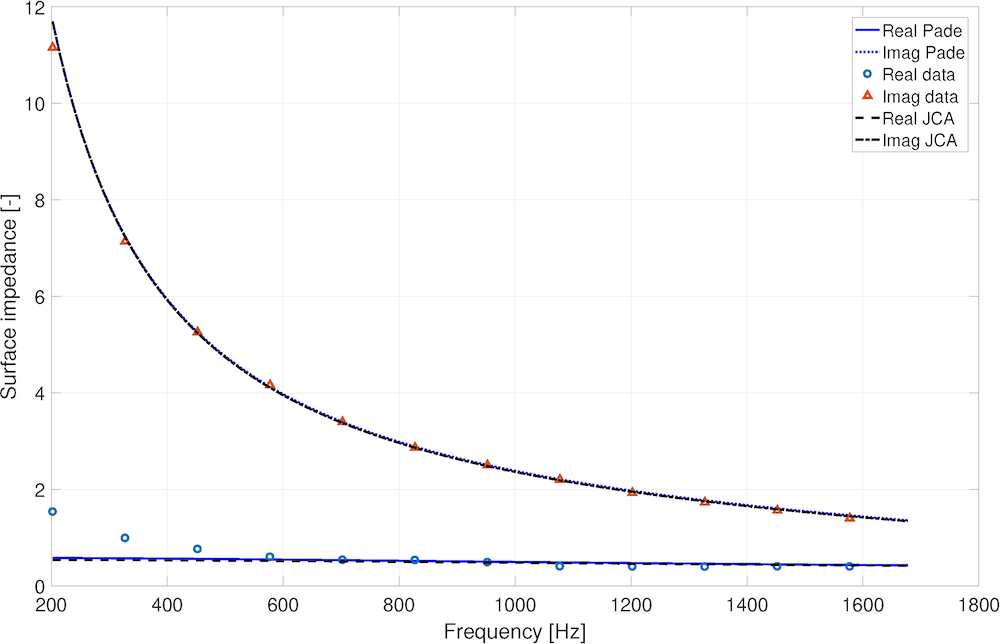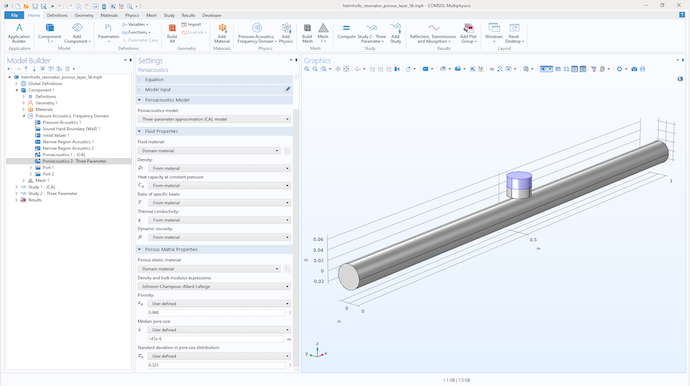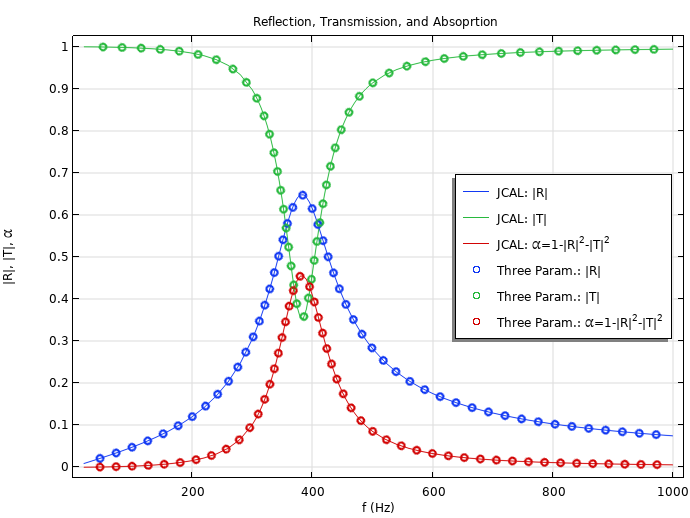# 使用 COMSOL® 模拟多孔介质的声学特性

2021年 1月 14日

### 6 参数 Johnson–Champoux–Allard–Lafarge 模型

COMSOL Multiphysics 软件包含一系列可以预测多孔介质声学特性的模型。在以往的应用中，声学模块提供的多孔介质声学功能（图1），使用了其中包含的Johnson-Champoux-Allard-Lafarge（JCAL）模型来进行预测，其结果被广泛引用（截至 2020 年 11 月 15 日，已有超过 2000 多个 Scopus 文摘和引文数据库引用）。

JCAL 模型最初于 1991 年被提出（参考文献1）。它需要 6 个非声学参数来预测材料孔隙中流体复杂的、随频率变化的动态密度：

(1)

\rho(\omega)=\frac{\rho_f \alpha_\infty}{\epsilon_p} \left[ 1+\frac{\sigma \epsilon_p}{i \alpha_\infty \rho_f \omega} \left( 1+\frac{4i \alpha ^{2}_\infty \mu \rho_f \omega}{\sigma^2 \Lambda^2 \epsilon^{2}_{p}}\right)^{1/2} \right]

(2)

C(\omega) = \frac{\epsilon_p}{\gamma P_0} \left[\gamma – \frac{\gamma-1}{1-\frac{i \sigma’ \epsilon_p}{\rho_f \alpha_\infty N_\textrm{Pr} \omega} \left(1+\frac{4i \alpha^{2}_{\infty}\mu \rho_f N_\textrm{Pr}\omega}{(\sigma’ \Lambda’ \epsilon_p)^2}\right)^{1/2}} \right]

COMSOL文档和由Matelys 运营的声学多孔介质材料设计（Acoustical Porous Material Recipes，APMR）网站中，对这些方程的物理含义进行了全面的解释。在上述公式中，控制多孔介质声学特性的关键非声学参数为：

1. 孔隙率， \epsilon_p
2. 黏性流阻，\sigma
3. 热流阻，\sigma’
4. 黏性特征长度，\Lambda
5. 热特性长度 \Lambda’
6. 曲折因子，\alpha_\infty

1. 流体的平衡密度，\rho_f
2. i = \sqrt{-1}
3. 声音的角频率，\omega
4. 流体的动态黏度，\mu
5. 普朗特数，N_\textrm{Pr}

(3)

(4)

r(\omega)=\frac{z_s(\omega)-1}{z_s(\omega)+1}

(5)

z_s(\omega)=z_b(\omega)\coth(ik_b(\omega)h)/(\rho_f c_0)

### 从 6 个参数到 3 个参数的 JCAL 模型

1. 孔隙率，\epsilon_p
2. 平均孔径，\bar{s}
3. 孔径标准偏差，\sigma_s

\Lambda = \bar{s}e^{-5/2(\sigma_s \text{log} 2)^2}
\Lambda’=\bar{s}e^{3/2(\sigma_s \text{log}2)^2}

\alpha_\infty=e^{4(\sigma_s\text{log}2)^2}.

### 在 COMSOL Multiphysics® 中选择 3 参数模型### 3 参数模拟的示例应用### 动手尝试

1. Champoux, Y. and Allard, J.-F., “Dynamic tortuosity and bulk modulus in air-saturated porous media”, Appl. Phys., vol. 70, no. 4, pp. 1975–1979, 1991. https://doi.org/10.1063/1.349482
2. Doutres, O., Salissou, Y., Atalla, N., Panneton, R. “Evaluation of the acoustic and non-acoustic properties of sound absorbing materials using a three microphone impedance tube”, Acoust., vol. 71, no. 6, pp. 506–509, 2010. http://dx.doi.org/10.1016/j.apacoust.2010.01.007
3. ISO 10534-2:1998, “Determination of sound absorption coefﬁcient and impedance in impedance tubes, Part 2: Transfer-function method”, International Organization for Standardization, Geneva, Switzerland, 1998. https://www.iso.org/standard/22851.html
4. Horoshenkov, K. V., “A Review of acoustical methods for porous material characterisation”, J. Acoust. Vib., vol. 22, vol. 1, pp. 92–103, 2017. https://doi.org/10.20855/ijav.2017.22.1455
5. Allard, J.-F. and Champoux, Y., “New empirical equations for sound propagation in rigid frame ﬁbrous materials”, Acoust. Soc. Am., vol. 91, no. 6, pp. 3346–3353, 1992. https://doi.org/10.1121/1.402824
6. Allard, J.-F., Henry, M. Tizianel, J., Kelders L. and Lauriks, W., “Sound propagation in air-saturated random packings of beads”, Acoust. Soc. Am., vol. 104, no. 4, pp. 2004–2007, 1998. https://doi.org/10.1121/1.423766
7. Perrot, C., Chevillotte, F., Hoang, M. T., Bonnet, G. BÃl’cot, F.-X., Gautron, L. and Duval, A., “Microstructure, transport, and acoustic properties of open-cell foam samples: Experiments and three-dimensional numerical simulations”, Appl. Phys., vol. 111, no. 014911, 2012. https://doi.org/10.1063/1.3673523
8. Horoshenkov, K. V., Groby, J.-P., and Dazel, O., “Asymptotic limits of some models for sound propagation in porous media and the assignment of the pore characteristic lengths”, Acoust. Soc. Am., vol. 139, no. 5, pp. 2463–2474, 2016. https://doi.org/10.1121/1.4947540
9. Horoshenkov, K. V., Hurrell, A. and Groby, J.-P., “A three-parameter analytical model for the acoustical properties of porous media”, vol. 145, no. 4, pp. 2512–25178, 2019. https://doi.org/10.1121/1.5098778

### 关于作者

Kirill Horoshenkov（FREng）是英国谢菲尔德大学（University of Shefﬁeld，UK）机械工程系的声学教授。他于 1989 年毕业于俄罗斯莫斯科无线电工程与自动化大学（Moscow University for Radioengineering and Automatics, Russia），并获得了电声与超声工程学硕士学位。1997 年，他获得了英国布拉德福德大学（University of Bradford，UK）的声学博士学位。他的研究包括室外声音传播、多孔介质的声学以及机器人的声学感应。由他带领EPSRC Programme Grant PipebotsEPSRC UK Acoustics Network 超过了 1000 名成员。他发表了超过 90 多篇经评审的期刊论文和相似数量的会议论文，拥有 10 项专利或专利申请（作者或合著者）。三项以他的名字或由他直接参与的专利已经被 Acoutechs 公司（由Armacell接管的声学材料制造商），Acoustic Sensing Technology 公司（对埋入式管道的声学检查）和nuron 公司（埋入式光纤电缆传感）进行了商业开发。